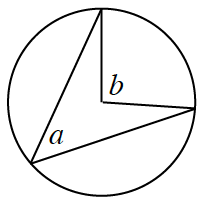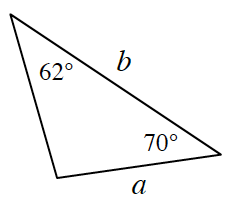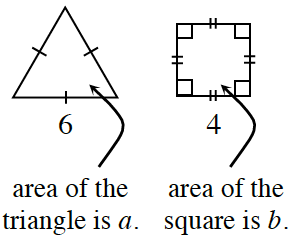### Home > CCG > Chapter 11 > Lesson 11.1.1 > Problem11-18

11-18.

For each geometric relationship below, determine whether $a$ or $b$ is larger, or if they are equal. Assume that the diagrams are not drawn to scale. If there is not enough information, explain what information is missing.

1.How does the central angle relate to the inscribed angle?

1.Find the measure of the last angle.
How do angles relate to the lengths of the sides?

Since the angle opposite side '$a$' is bigger, '$a$' must be larger than '$b$'.

1.Use the given information to find the areas.
Which is bigger?

$a = 15.6 \; \text{units}^2$
$b = 16 \; \text{units}^2$